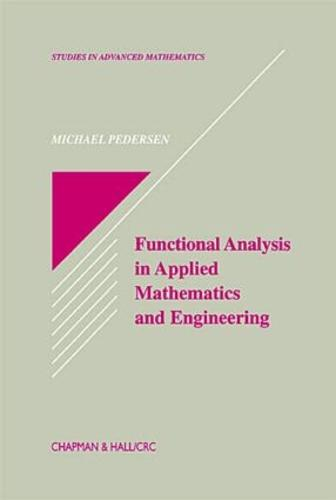•# Functional Analysis in Applied Mathematics and Engineering - Studies in Advanced Mathematics 31 (Hardback)

(author)
£140.00
Hardback 312 Pages / Published: 29/09/1999
• We can order this

Usually dispatched within 3 weeks

Presenting excellent material for a first course on functional analysis , Functional Analysis in Applied Mathematics and Engineering concentrates on material that will be useful to control engineers from the disciplines of electrical, mechanical, and aerospace engineering.

This text/reference discusses:
rudimentary topology
Banach's fixed point theorem with applications
L^p-spaces
density theorems for testfunctions
infinite dimensional spaces
bounded linear operators
Fourier series
open mapping and closed graph theorems
compact and differential operators
Hilbert-Schmidt operators
Volterra equations
Sobolev spaces
control theory and variational analysis
Hilbert Uniqueness Method
boundary element methods

Functional Analysis in Applied Mathematics and Engineering begins with an introduction to the important, abstract basic function spaces and operators with mathematical rigor, then studies problems in the Hilbert space setting. The author proves the spectral theorem for unbounded operators with compact inverses and goes on to present the abstract evolution semigroup theory for time dependent linear partial differential operators. This structure establishes a firm foundation for the more advanced topics discussed later in the text.

Publisher: Taylor & Francis Inc
ISBN: 9780849371691
Number of pages: 312
Weight: 617 g
Dimensions: 235 x 159 x 24 mm